Seleccionar página

Abstract:
Multiplicative functions play an important role in Analytic Number Theory. By multiplicative we mean that

whenever positive integers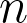and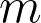are coprime. Since the prime powers are the building blocks of multiplication, we have that a multiplicative function is completely determined by its values at

these powers.

An important example that will be discussed in this talk is the Möbius function which is defined to be at primes

and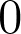at powers bigger than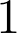of primes. For example, while . When we consider the partial sums of the Möbius function, we can see this as an “arithmetic random walk”, and naturally we can ask about the size of the fluctuations of this walk. Just as in the simple random walk in Probability theory, we expect square-root cancellation for these sums, but it turns out that to prove this is so difficult as to establish the Riemann Hypothesis (RH). Inspired by the square-root cancellation in the i.i.d. simple random walk, this equivalence of the sums of Möbius with RH led many authors to investigate statistical properties of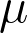. An important work is that of Wintner in the 40’s. He considered a question proposed by Lévy, and investigated the model of a

random multiplicative function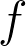, which is nothing more than a multiplicative function where at primes,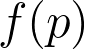is an i.i.d. sequence of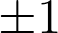with probability 1/2 each. Wintner proved almost sure square-root cancelation for this multiplicative random walk, and later many authors investigated this problem from different perspectives. In this talk, my plan is to present what is known in the literature about these random functions and also to present my joint contribution at different papers with Vladas Sidoravicius, and with Winston Heap and Jing Zhao.

Date: Aug 17, 2022 at 16:15:00 h